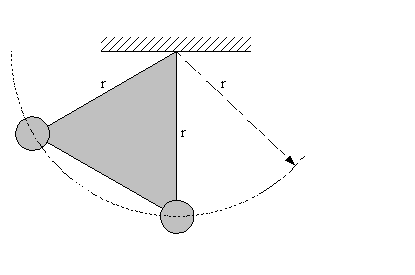Hi,
I am reviewing a problem with the associated solution and there is something i don t understand.
Imagine a triangle with vertices l, masses m are attached to the two end of the vertices and on the top end vertice there is a pivot so that the triangle can swing. We start with the triangle at rest and one of the rod is vertical.
Question: what is the accelerations of the masses (the two following words are in italics) just after it is released?
What i don t understand is the following:
"since v=0 at the start , the radial accelerations are zero"(quote from the solution). And then, the problem is treated as there is no radial acceleration at all, while considering a tangential acceleration. How can we consider a tangential acceleration without a radial/centripetal acceleration?
If this is possible, could you explain me and clarify with an example?
Thank you.

gneill
Mentor
Hi,
I am reviewing a problem with the associated solution and there is something i don t understand.
Imagine a triangle with vertices l, masses m are attached to the two end of the vertices and on the top end vertice there is a pivot so that the triangle can swing. We start with the triangle at rest and one of the rod is vertical.
Question: what is the accelerations of the masses (the two following words are in italics) just after it is released?
What i don t understand is the following:
"since v=0 at the start , the radial accelerations are zero"(quote from the solution). And then, the problem is treated as there is no radial acceleration at all, while considering a tangential acceleration. How can we consider a tangential acceleration without a radial/centripetal acceleration?
If this is possible, could you explain me and clarify with an example?
Thank you.

So you have something that looks like this?If the 'rods' forming the triangle are of fixed length, then the masses are constrained to follow the circular arc indicated, and there's no change in length of r possible. So no radial acceleration. This is not to say that centripetal forces will not happen, just that they cannot make the masses accelerate due to the fixed lengths of the triangle.

It's like a book lying on a table; There's a gravitational force acting on it, and g is still in business, but still the book does not move downwards.

You might consider treating the object as a physical pendulum and determine its angular acceleration first.